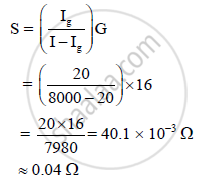HSC Science (Electronics) 12th Board ExamMaharashtra State Board
Share

# A Galvanometer Has a Resistance of 16ω. It Shows Full Scale Deflection, When a Current of 20 Ma is Passed Through It. the Only Shunt Resistance Available is 0.06 Which is Not Appropriate to Convert a Galvanometer into an Ammeter. How Much Resistance Should Be Connected in Series with the Coil of Galvanometer, So that the Range of Ammeter is 8 a - HSC Science (Electronics) 12th Board Exam - Physics

#### Question

A galvanometer has a resistance of 16Ω. It shows full scale deflection, when a current of 20 mA is passed through it. The only shunt resistance available is 0.06  which is not appropriate to convert a galvanometer into an ammeter. How much resistance should be connected in series with the coil of galvanometer, so that the range of ammeter is 8 A?

#### Solution

G = 16Ω, Ig = 20 mA, I = 8A, S = ?A resistance of ≈ 0.04 Ω should be connected in series with the coil of the galvanometer.

Is there an error in this question or solution?

#### APPEARS IN

2012-2013 (October) (with solutions)
Question 5.2 | 7.00 marks

#### Video TutorialsVIEW ALL 

Solution A Galvanometer Has a Resistance of 16ω. It Shows Full Scale Deflection, When a Current of 20 Ma is Passed Through It. the Only Shunt Resistance Available is 0.06 Which is Not Appropriate to Convert a Galvanometer into an Ammeter. How Much Resistance Should Be Connected in Series with the Coil of Galvanometer, So that the Range of Ammeter is 8 a Concept: Moving Coil Galvanometer.
S